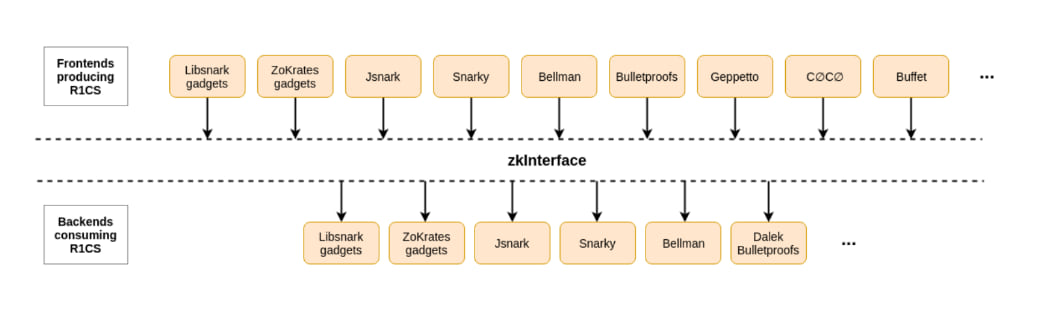## bin+lib zkinterface

An implementation of zkInterface

### 18 stable releases

 1.3.4 Mar 8, 2021 Feb 22, 2021 Oct 21, 2020 Aug 19, 2020 May 10, 2019

#73 in #zero-knowledge

Used in 6 crates

140KB
3K SLoC

# zkInterface, a standard tool for zero-knowledge interoperability

zkInterface is a standard tool for zero-knowledge interoperability between different ZK DSLs, gadget libraries, and proving systems. The zkInterface project was born in the ZKProof community.

## IntroductionzkInterface is specification and associated tools for enabling interoperability between implementations of general-purpose zero-knowledge proof systems. It aims to facilitate interoperability between zero knowledge proof implementations, at the level of the low-constraint systems that represent the statements to be proven. Such constraint systems are generated by frontends (e.g., by compilation from higher-level specifications), and are consumed by cryptographic backends which generate and verify the proofs. The goal is to enable decoupling of frontends from backends, allowing application writers to choose the frontend most convenient for their functional and development needs and combine it with the backend that best matches their performance and security needs.

The standard specifies the protocol for communicating constraint systems, for communicating variable assignments (for production of proofs), and for constructing constraint systems out of smaller building blocks (gadgets). These are specified using language-agnostic calling conventions and formats, to enable interoperability between different authors, frameworks and languages. A simple special case is monolithic representation of a whole constraint system and its variable assignments. However, there are a need for more richer and more nuanced forms of interoperability:

• Precisely-specified statement semantics, variable representation and variable mapping
• Witness reduction, from high-level witnesses to variable assignments
• Gadgets interoperability, allowing components of constraint systems to be packaged in reusable and interoperable form
• Procedural interoperability, allowing execution of complex code to facilitate the above

# Examples

zkInterface does not force a serialization method, it should be provided by the user - `serialize_small()` in this example.

Create a `CircuitHeader`

``````use zkinterface::producers::examples::{serialize_small, NEG_ONE};
use zkinterface::KeyValue;

let (x,y,zz) = (3,4,25);

// variables ids 1,2 and 3 are used as instances variables
instance_variables: zkinterface::Variables {
variable_ids: vec![1, 2, 3],  // x, y, zz
values: Some(serialize_small(&[x, y, zz])),
},
free_variable_id: 6,
field_maximum: Some(serialize_small(&[NEG_ONE])),
configuration: Some(vec![
KeyValue::from(("Name", "example")),
]),
};
``````

``````let (x,y) = (3,4);

use zkinterface::producers::examples::serialize_small;

//variables ids 4 and 5 are used as witness variables
let witness = zkinterface::Witness {
assigned_variables: zkinterface::Variables {
variable_ids: vec![4, 5], // xx, yy
values: Some(serialize_small(&[
x * x, // var_4 = xx = x^2
y * y, // var_5 = yy = y^2
])),
}
};
``````

Create a `ConstraintSystem` from an R1CS vector

``````let constraints_vec: &[((Vec<u64>, Vec<u8>), (Vec<u64>, Vec<u8>), (Vec<u64>, Vec<u8>))] = &[
// (A ids values)  *  (B ids values)  =  (C ids values)
((vec!, vec!), (vec!, vec!), (vec!, vec!)), // x * x = xx
((vec!, vec!), (vec!, vec!), (vec!, vec!)), // y * y = yy
((vec!, vec!), (vec![4, 5], vec![1, 1]), (vec!, vec!)), // 1 * (xx + yy) = z
];

let constraints = zkinterface::ConstraintSystem::from(constraints_vec);
``````

## The Statement Builder

zkInterface provides a `StatementBuilder` to assists with constructing and storing a statement in zkInterface format.

`````` use zkinterface::{StatementBuilder, Sink, WorkspaceSink, CircuitHeader, ConstraintSystem, Witness};

// Create a workspace where to write zkInterafce files.
let sink = WorkspaceSink::new("local/test_builder").unwrap();
let mut builder = StatementBuilder::new(sink);

// Use variables, construct a constraint system, and a witness.
let var_ids = builder.allocate_vars(3);
let cs = ConstraintSystem::default();
let witness = Witness::default();

builder.push_witness(witness).unwrap();
builder.push_constraints(cs).unwrap();
``````

## The Simulator

zkInterface provides a Simulator to check entire constraint system and output what constraints are violated, if any.

`````` # use zkinterface::producers::examples::{example_circuit_header, example_witness, example_constraints};
use zkinterface::consumers::simulator::Simulator;

pub fn simulate() -> zkinterface::Result<()> {
let witness = example_witness();
let cs = example_constraints();

let mut simulator = Simulator::default();
simulator.ingest_witness(&witness)?;
simulator.ingest_constraint_system(&cs)?;
Ok(())
}
``````

## The Validator

zkInterface provides a Validator to check the syntax and the semantics of the provided parameters and return a list of violations, if any.

`````` # use zkinterface::producers::examples::{example_circuit_header, example_witness, example_constraints};
use zkinterface::consumers::validator::Validator;

let witness = example_witness();
let constraints = example_constraints();

let mut validator = Validator::new_as_prover();
validator.ingest_witness(&witness);
validator.ingest_constraint_system(&constraints);

let violations = validator.get_violations();
if violations.len() > 0 {
eprintln!("Violations:\n- {}\n", violations.join("\n- "));
}
``````

In addition to the library, a CLI tool is provided. The CLI tool can execute the following commands:

• `zkif example` Create example statements.
• `zkif cat` Write .zkif files to stdout.
• `zkif to-json` Convert to JSON on a single line.
• `zkif to-yaml` Convert to YAML.
• `zkif explain` Print the content in a human-readable form.
• `zkif validate` Validate the format and semantics of a statement, as seen by a verifier.
• `zkif simulate` Simulate a proving system as prover by verifying that the statement is true.
• `zkif stats` Calculate statistics about the circuit.
• `zkif clean` Clean workspace by deleting all *.zkif files in it.

~4–15MB
~189K SLoC